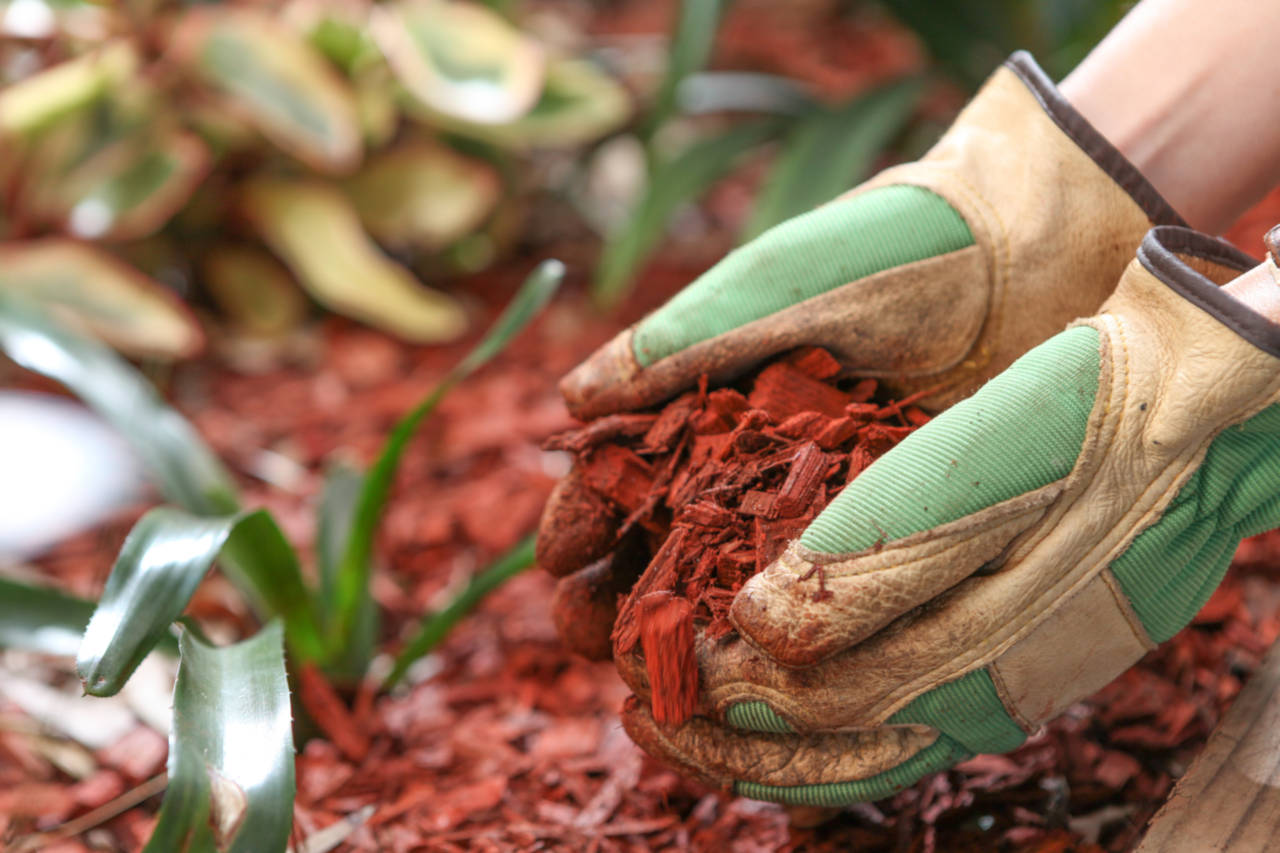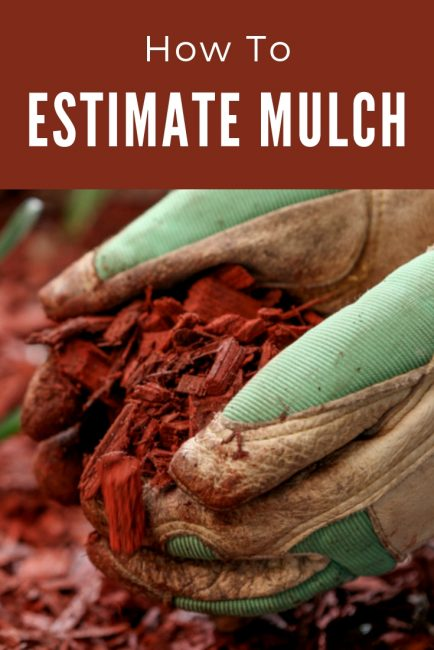# Mulch Calculator – How Much Do You Need?

Calculate how much mulch you need by entering the size of the landscaping area and the depth of fill. Get results in cubic feet, cubic yards, and the number of bags of mulch needed.

Find area using our square footage calculator

## Mulch Needed:

Cubic yards

Cubic feet
Number of Mulch Bags

2 cu. ft. bags

3 cu. ft. bags
Learn how we calculated this below

## How Much Mulch Do You Need?

Adding mulch is a great way to refresh a landscaping bed and prevent weed growth.

Adding mulch is a pretty easy project, but as you begin, you might be wondering how much mulch you need.

You’re in luck! Follow along, and we’ll show you how to calculate how much mulch you need for your landscaping project.

The easiest way to calculate mulch coverage is to use the calculator above since it takes all the math and guesswork out of the process.### Steps to Calculate Mulch

If you want to do the calculations yourself, you can estimate material in a few easy steps.

#### Step One: Measure the Landscaping Space

The first step to calculating how much mulch you need is to measure the space you want to cover. Use a tape measure to measure the length and width of the space.

Next, measure the depth of the mulch bed. We recommend a 3″ depth for an average mulch bed, but 2″ or 4″ would be just fine as well.

Ensure all measurements are in the same unit of measure; the calculations will be easiest if all measurements are in feet.

#### Step Two: Calculate the Volume of the Landscaping Space

Once you have measured the space, it’s time to calculate the volume.

Multiply the length times the width to find the area of the space. If the landscape bed is complex, then split it up into manageable sections and measure each separately.

For landscape beds with portions that aren’t a simple rectangle, you can use an area calculator to estimate the area of more complex shapes.

Then, multiply the area by the depth. Remember to keep all measurements in the same unit of measure, so if the width and length are in feet, then multiply the area by the depth in feet.

##### Volume Formula

For an area that is a standard rectangle, you can use the following formula to calculate the volume.

volume = length × width × depth

#### Step Three: Convert the Volume to the Unit Needed

The final step to calculating how much mulch you need is to convert the volume measurement to the unit of measure that the mulch is sold by if it isn’t already in the same unit of measure.

Mulch is usually sold in bulk by the cubic yard at most landscape suppliers.

When calculating volume, if all the measurements used are in feet, then the volume is in cubic feet. If the measurements were in inches, then the volume is cubic inches.

#### How Many Cubic Feet in a Yard of Mulch?

There are 27 cubic feet in one yard of mulch. Convert cubic feet to cubic yards by dividing by 27.

If you measured in inches, first divide the cubic inches measurement by 1,728 to get cubic feet, then divide again by 27 to get cubic yards.

#### Mulch Formulas

cubic feet = cubic inches ÷ 1,728

cubic yards = cubic feet ÷ 27

You can also use our cubic inches to cubic feet or cubic feet to cubic yards calculators.

## How Many Bags Do You Need?

When buying it at the hardware store, home center, or local market, it’s usually sold by the bag. Most bags hold two-cubic feet of mulch, but in some cases they hold 3 cubic feet.

### How Many Bags of Mulch in a Yard?

So you’ve followed the steps above and found how many yards you need, but how many bags of mulch are in a cubic yard? There are 14 (2 cu ft) bags in one cubic yard, or 9 (3 cu ft) bags.

Number of bags of mulch equivalent to a cubic yard.
cubic yards 2 cu ft bags 3 cu ft bags
1 cu yd 14 bags 9 bags
2 cu yds 27 bags 18 bags
3 cu yds 41 bags 27 bags
4 cu yds 54 bags 36 bags
5 cu yds 68 bags 45 bags

## How Much Does a Yard of Mulch Cover?

At three inches deep, one yard of mulch will cover just over 100 square feet of landscaping. Refer to the table below to see how much a yard will cover at various depths.

Coverage of one cubic yard of mulch at various depths
mulch depth coverage per yard
1 inch 324 sq ft
2 inches 162 sq ft
3 inches 108 sq ft
4 inches 81 sq ft
5 inches 64 sq ft

## How Much Does it Weigh?

If you’re hauling mulch yourself, it’s important to understand how much it weighs so you can plan to transport it safely.

### How Much Does a Yard of Mulch Weigh?

Depending on the moisture content, a yard of mulch weighs 400 – 800 pounds. The type of material and the amount of moisture it contains will impact its weight.

### How Much Does a Bag of Mulch Weigh?

On average, one 2 cu ft bag of mulch weighs 20 pounds. The moisture content and type of material will also impact the weight of each bag.

## What Type of Mulch Should You Use?

Before purchasing mulch, do some research on the best type for your project. Backyard Boss explains that there are several types available, including standard garden, rubber, bark, hardwood, hemlock, and mulched leaves.

Each type has a different look, so spend some time to find the right variety to complement your home and landscaping. Different types also cost more than others, which may be an important factor in your decision.

Check out our soil calculator to calculate soil for a garden bed and use our cubic yardage calculator to estimate other landscaping materials.## References

1. Backyard Boss, Everything You Ever Needed to Know About Mulch, https://www.backyardboss.net/what-is-mulch/